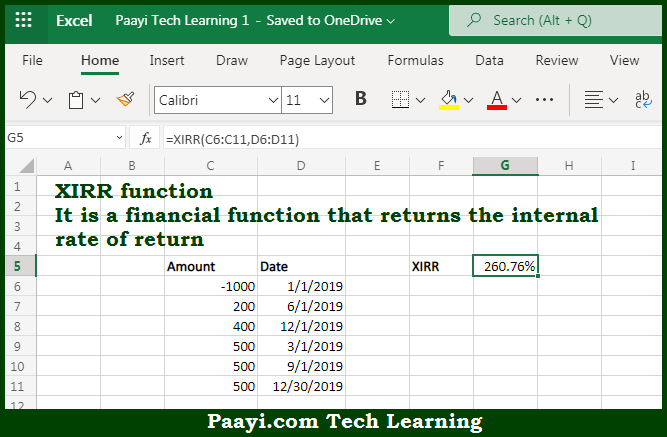# Learn How to Use Microsoft Excel XIRR Function

Written by | 0 Comments | 588 Views

In this article, you will learn how to use the Microsoft Excel XIRR function and its prime function in Microsoft Excel. You will also get to know the Microsoft Excel XIRR function return value and syntax with the help of some examples.

Microsoft Excel XIRR Function

The main purpose of the Microsoft Excel XIRR function is to calculate the internal rate of return for irregular cash flows. That implies, with the help of the XIRR function you can able to return the internal rate of return that is IRR, for a series of cash flows that occur at irregular intervals. So, with the help of the XIRR function, you can able to calculate the internal rate of return for irregular cash flows.

Return Value of XIRR Function

The return value will be the calculated return in the form of a percentage.

Syntax of XIRR Function

=XIRR(values, dates, [guess])

Where the arguments:

• values: This is an array or reference to cells containing the cash flows.
• dates: The are the dates corresponding to the cash flows.
• guess: This is an estimate for expected IRR, and Default is .1 or 10% (optional).

How to Use Microsoft Excel XIRR Function?So we know that Microsoft Excel XIRR function you can able to calculate the internal rate of return for irregular cash flows. That implies, with the help of the XIRR function you can able to return the internal rate of return that is IRR, for a series of cash flows that occur at irregular intervals. So, with the help of the XIRR function, you can able to calculate the internal rate of return for irregular cash flows.